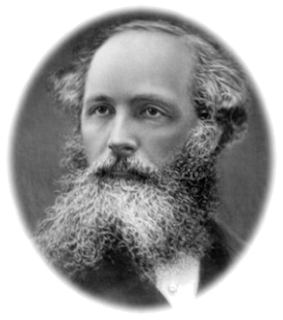Talking about magnetic field, we have three relevant physical quantities - magnetic field $$\vec{H}$$, magnetic induction field $$\vec{B}$$ and magnetization field $$\vec{M}$$. It is straightforward to understand $$\vec{M}$$ - when applying magnetic field (here, by ‘magnetic field’, we mean something in general, but not specifically refer to $$\vec{H}$$), we use $$\vec{M}$$ to characterize how much the matter in question is magnetized. However, concerning $$\vec{H}$$ and $$\vec{B}$$, it seems that both are describing some sort of ‘strength’ of magnetic field (again, we mean ‘magnetic field’ in general. The same applies below until we become specific about what we mean by ‘magnetic field’). But why do we have two quantities here to describe the ‘same’ thing? The answer is - they are not the same thing, as described by $$\vec{H}$$ and $$\vec{B}$$, respectively. Fundamentally, this goes back to the foundation of the electromagnetic theory - specifically, we mean the two historical discoveries. One is the finding that an electric current creates a magnetic field, by Oersted. The other is the discovery by Faraday that, in brief, a changing magnetic field will create electromotive force across an electrical conductor. The first one describes how a magnetic field can be generated from a current. Starting from such a discovery, if we are going to define a quantity to describe magnetic field, such a quantity will be bounded to electrical current, i.e. such a defined quantity for describing magnetic field can be purely derived from electrical current - this is the quantity $$\vec{H}$$, to which we give the name of magnetic filed (we now become specific about what we mean by ‘magnetic field’). Concerning the second discovery, we also have a correspondingly defined quantity to describe the associated magnetic field (here, we go back to the general meaning of saying ‘magnetic field’, temporarily) – the quantity we define as $$\vec{B}$$, to which we give the name of magnetic induction field. Different from $$\vec{H}$$, the quantity $$\vec{B}$$ serves as the ‘source’ of electric field, i.e. $$\vec{B}$$ is considered to induce electric field (thus given the name magnetic induction field) and therefore $$\vec{B}$$ does not have to originate itself purely from electrical current. In another word, when we talk about the induction of electric field from magnetic ‘stuff’, we mean every aspect of the magnetic ‘stuff’, including both that generated from electrical current (i.e. the magnetic field $$\vec{H}$$) and the magnetization effect of matter (i.e., the magnetization field $$\vec{M}$$). After all, the following relation summarizes the discussion above,

$\vec{B} = \mu_0(\vec{H} + \vec{M})$

The two discoveries mentioned above were further summarized into Maxwell’s equation in matter (i.e., non-vacuum), respectively,

$\nabla \times \vec{E} = -\frac{1}{c}\frac{\partial \vec{B}}{\partial t}$ $\nabla \times \vec{H} = \frac{1}{c}(4\pi \vec{J}_f + \frac{\partial \vec{D}}{\partial t})$

In summary, $$\vec{H}$$ can be thought of as being purely coming from electrical current, whereas $$\vec{B}$$ covers both the part originating from electrical field and that from matter magnetization (i.e., a comprehensive ‘magnetic field’) – that’s why the Gauss’s law for magnetism is summarized as $$\nabla \cdot \vec{B} = 0$$, where the comprehensive quantity $$\vec{B}$$ is used instead of $$\vec{H}$$.